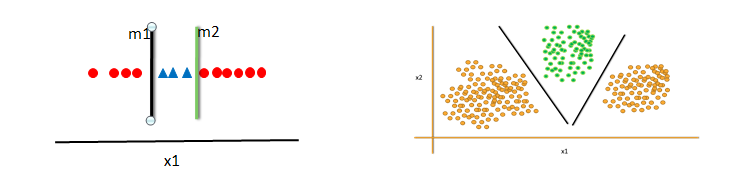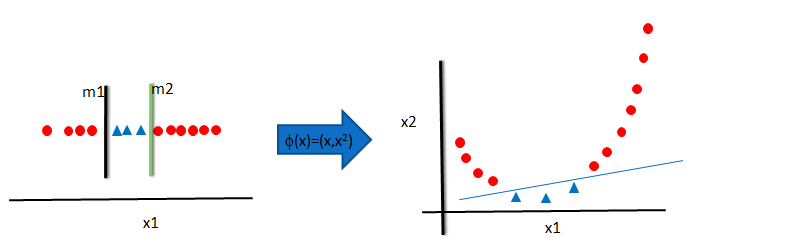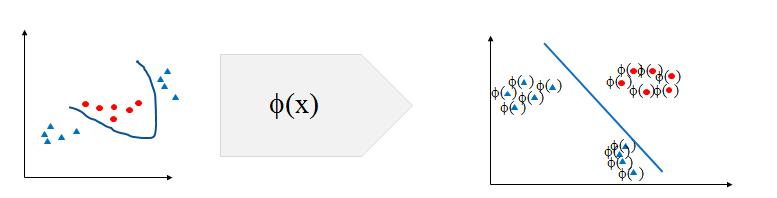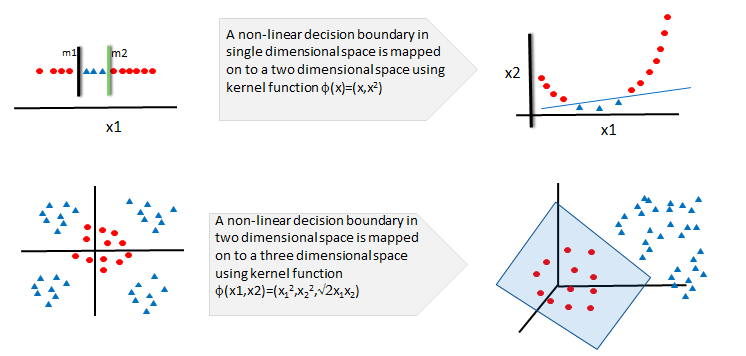• No products in the cart.

# 203.6.5 The Non-Linear Decision Boundary

## The Non-Linear Decision Boundary

In previous section, we studied about Building SVM model in R

• In the above examples we can clearly see the decision boundary is linear
• SVM works well when the data points are linearly separable
• If the decision boundary is non-liner then SVM may struggle to classify
• Observe the below examples, the classes are not linearly separable
• SVM has no direct theory to set the non-liner decision boundary models.### Mapping to Higher Dimensional Space

• The original maximum-margin hyperplane algorithm proposed by Vapnik in 1963 constructed a linear classifier.
• To fit a non liner boundary classier, we can create new variables(dimensions) in the data and see whether the decision boundary is linear.
• In 1992, Bernhard E. Boser, Isabelle M. Guyon and Vladimir N. Vapnik suggested a way to create nonlinear classifiers by applying the kernel trick
• In the below example, A single linear classifier is not sufficient
• Lets create a new variable $x2=(x1)^2$. In the higher dimensional space
• We can clearly see a possibility of single linear decision boundary
• This is called kernel trick### Kernel Trick

• We used a function f(x)=(x,$x^2$) to transform the data x into a higher dimensional space.
• In the higher dimensional space, we could easily fit a liner decision boundary.
• This function f(x) is known as kernel function and this process is known as kernel trick in SVM• Kernel trick solves the non-linear decision boundary problem much like the hidden layers in neural networks.
• Kernel trick is simply increasing the number of dimensions. It is to make the non-linear decision boundary in lower dimensional space as a linear decision boundary, in higher dimensional space.
• In simple words, Kernel trick makes the non-linear decision boundary to linear (in higher dimensional space)### Kernel Function Examples

Name Function Type problem
Polynomial Kernel $(x_i^t x_j +1)^q$ q is degree of polynomial Best for Image processing
Sigmoid Kernel $tanh(ax_i^t x_j +k)$ k is offset value Very similar to neural network
Gaussian Kernel $e^(||x_i – x_j||^2/2 \sigma^2)$ No prior knowledge on data
Linear Kernel $1+x_i x_j min(x_i , x_j) – \frac{(x_i + x_j)}{2} min(x_i , x_j)^2 + \frac{min(x_i , x_j)^3}{3}$ Text Classification
Laplace Radial Basis Function (RBF) $e^(-\lambda ||x_i – x_j||) , \lambda >= 0$ No prior knowledge on data
• There are many more kernel functions.

### Choosing the Kernel Function

• Probably the most tricky part of using SVM.
• The kernel function is important because it creates the kernel matrix, which summarizes all the data
• There is no proven theory for choosing a kernel function for any given problem. Still there is lot of research going on.
• In practice, a low degree polynomial kernel or RBF kernel with a reasonable width is a good initial try
• Choosing Kernel function is similar to choosing number of hidden layers in neural networks. Both of them have no proven theory to arrive at a standard value.
• As a first step, we can choose low degree polynomial or radial basis function or one of those from the list.

The next post is about a  practice session on Kernel Non-linear Classifier.

### 0 responses on "203.6.5 The Non-Linear Decision Boundary"

Statinfer Software Solutions LLP

Software Technology Parks of India,
NH16, Krishna Nagar, Benz Circle,